# Law of Sines

The Law of Sines is the relationship between the sides and angles of non-right (oblique) triangles .  Simply, it states that the ratio of the length of a side of a triangle to the sine of the angle opposite that side is the same for all sides and angles in a given triangle.

In $\Delta ABC$ is an oblique triangle with sides $a,b$ and $c$ , then $\frac{a}{\mathrm{sin}A}=\frac{b}{\mathrm{sin}B}=\frac{c}{\mathrm{sin}C}$ .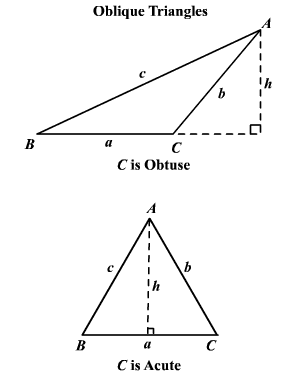To use the Law of Sines you need to know either two angles and one side of the triangle (AAS or ASA) or two sides and an angle opposite one of them (SSA).  Notice that for the first two cases we use the same parts that we used to prove congruence of triangles in geometry but in the last case we could not prove congruent triangles given these parts.  This is because the remaining pieces could have been different sizes.  This is called the ambiguous case and we will discuss it a little later.

Example 1: Given two angles and a non-included side (AAS).

Given $\Delta ABC$ with $m\angle A=30°$ , $m\angle B=20°$ and $a=45$ m.  Find the remaining angle and sides.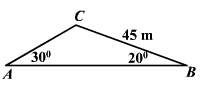The third angle of the triangle is

$m\angle C=180°-m\angle A-m\angle B=180°-30°-20°=130°$

By the Law of Sines,

$\frac{45}{\mathrm{sin}30°}=\frac{b}{\mathrm{sin}20°}=\frac{c}{\mathrm{sin}130°}$

By the Properties of Proportions

and

Example 2: Given two angles and an included side (ASA).

Given $m\angle A=42°$ , $m\angle B=75°$ and $c=22$ cm.  Find the remaining angle and sides.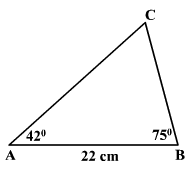The third angle of the triangle is:

$m\angle C=180°-m\angle A-m\angle B=180°-42°-75°=63°$

By the Law of Sines,

$\frac{a}{\mathrm{sin}42°}=\frac{b}{\mathrm{sin}75°}=\frac{22}{\mathrm{sin}63°}$

By the Properties of Proportions

and

## The Ambiguous Case

If two sides and an angle opposite one of them are given, three possibilities can occur.

(1) No such triangle exists.

(2) Two different triangles exist.

(3) Exactly one triangle exists.

Consider a triangle in which you are given $a,b$ and $A$ .  (The altitude $h$ from vertex $B$ to side $\stackrel{¯}{AC}$ , by the definition of sines is equal to $b\mathrm{sin}A$ .)

(1) No such triangle exists if $A$ is acute and $a or $A$ is obtuse and $a\le b$ .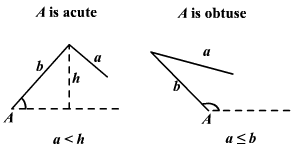(2) Two different triangles exist if $A$ is acute and $h .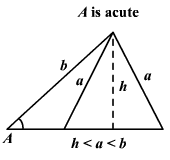(3) In every other case, exactly one triangle exists.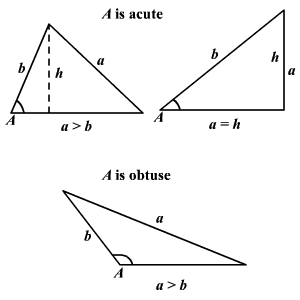Example 1: No Solution Exists

Given $a=15,b=25$ and $m\angle A=80°$ .  Find the other angles and side.

$h=b\mathrm{sin}A=25\mathrm{sin}80°\approx 24.6$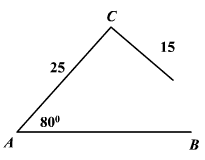Notice that $a . So it appears that there is no solution.  Verify this using the Law of Sines.

$\begin{array}{l}\frac{a}{\mathrm{sin}A}=\frac{b}{\mathrm{sin}B}\\ \frac{15}{\mathrm{sin}80°}=\frac{25}{\mathrm{sin}B}\\ \mathrm{sin}B=\frac{25\mathrm{sin}80°}{15}\approx 1.641>1\end{array}$

This contrasts the fact that the $-1\le \mathrm{sin}B\le 1$ .  Therefore, no triangle exists.

Example 2: Two Solutions Exist

Given $a=6,b=7$ and $m\angle A=30°$ .  Find the other angles and side.

$h=b\mathrm{sin}A=7\mathrm{sin}30°=3.5$

$h therefore, there are two triangles possible.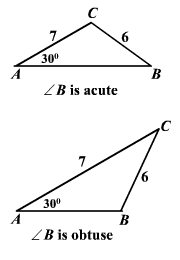By the Law of Sines, $\frac{a}{\mathrm{sin}A}=\frac{b}{\mathrm{sin}B}$

$\mathrm{sin}B=\frac{b\mathrm{sin}A}{a}=\frac{7\mathrm{sin}30°}{6}\approx 0.5833$

There are two angles between $0°$ and $180°$ whose sine is approximately 0.5833, are $35.69°$ and $144.31°$ .

Example 3: One Solution Exists

Given $a=22,b=12$ and $m\angle A=40°$ .  Find the other angles and side.

$a>b$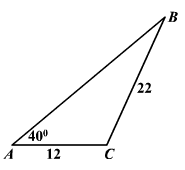By the Law of Sines, $\frac{a}{\mathrm{sin}A}=\frac{b}{\mathrm{sin}B}$

$\begin{array}{l}\mathrm{sin}B=\frac{b\mathrm{sin}A}{a}=\frac{12\mathrm{sin}40°}{22}\approx 0.3506\\ B\approx 20.52°\end{array}$

$B$ is acute.

$m\angle C=180°-m\angle A-m\angle B=180°-40°-20.52°=29.79°$

By the Law of Sines,

$\begin{array}{l}\frac{c}{\mathrm{sin}119.48°}=\frac{22}{\mathrm{sin}40°}\\ c=\frac{22\mathrm{sin}119.48°}{\mathrm{sin}40°}\approx 29.79\end{array}$

If we are given two sides and an included angle of a triangle or if we are given $3$ sides of a triangle, we cannot use the Law of Sines because we cannot set up any proportions where enough information is known.  In these two cases we must use the Law of Cosines .